# -4- Compute Confidence Interval

Let's assume you have planned a two-sided 3-stage Pocock design with 80% power.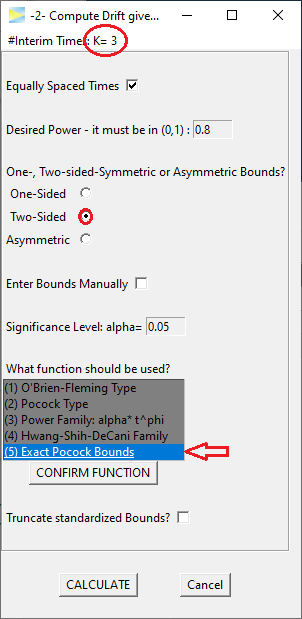The resulting design requires a drift of about 3.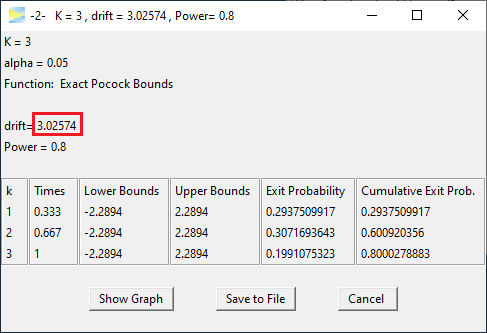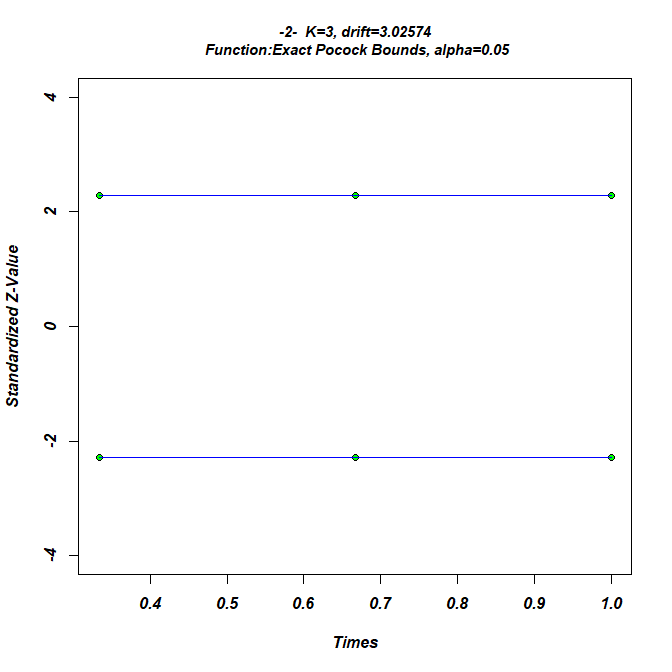During the study you perform the interim analysis as planned when about 67% of all samples were collected and obtain a standardized effect size of 2.5, exceeding the critical bound (2.2894) so that you can abort the study and reject H0.

To compute the confidence interval at this point, open option -4- of GroupSeq and enter the values as shown below.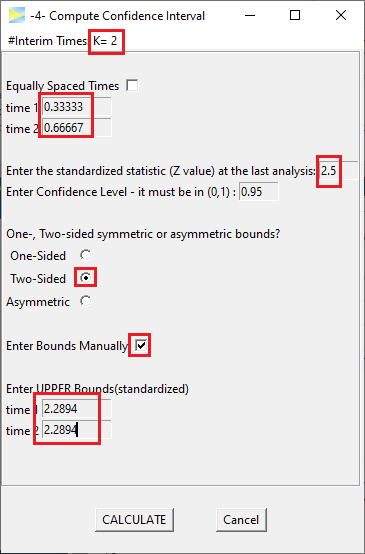Hitting CALCULATE yields the following.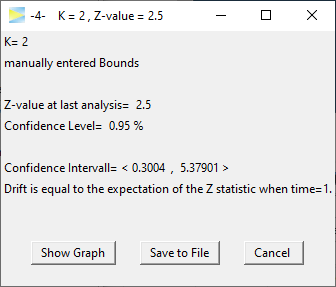So for this study, the resulting confidence interval of the standardized effect is (0.30, 5.38).

Depending on the underlying data distribution and applied test statistic this will be "back-calculated" to obtain the confidence interval for the value of interest (e.g. mean difference for some normally distributed data or difference in proportions of some binomially distributed data).